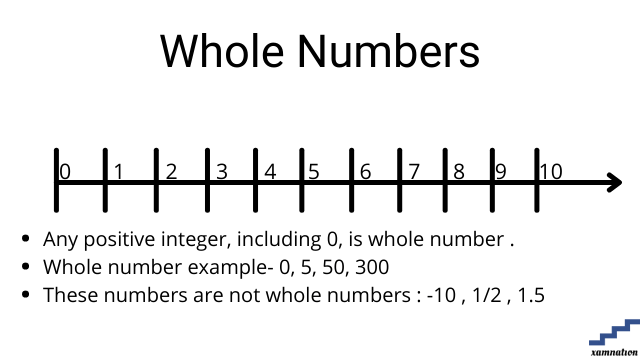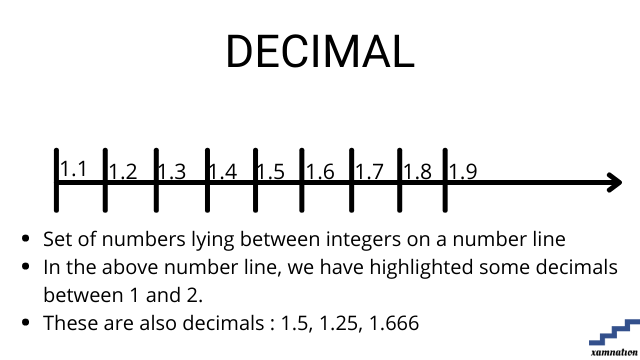# Maths for Grade 6 – Number System | what is whole number | what is Integer | what is Decimal

Number system tutorial

Number system is system of representing numbers in mathematics. Students deal with various types of numbers for solving mathematics formulas and calculation, data processing and handling complex topics like algebra and geometry.

Are you stuck in number system problem?

You can reach out to us for your math doubts and problem; our math tutors will help you in solving your doubts and take one-one math sessions online. Reach out to us on info@xamnation.com , and our qualified math tutors for grade 6 and above will be glad to help you.

## What is Whole Number?

### Definition of whole number

• Any positive integer (including 0) on the number line (up to infinity) is called whole number.
• It is positive number or 0
• It cannot be fraction, decimal or negative number### Examples of whole number

• 0 is a whole number
• 5, 10, 500, 3500 are whole numbers
• -5, 1.6 , 1/3 are not whole numbers

Practice question on whole number

Can you find whether below mentioned numbers are whole or not?

• 10
• 5
• 10/13
• -1.1
• -2/3
• 0
• 305

Send your solution of finding whole numbers to info@xamnation.com , and know detailed answer of this problem.

## What is Integer?

### Definition of Integer

• Any number on the number line (negative, positive or zero) is called Integer.
• Integer are both positive and negative
• Integers cannot be fraction and decimal### Examples of Integer

• 0 is a Integer
• 5, 10, 500, 3500 are Integer
• -5, -20, -45 are Integer
• 6 , 1/3 are not Integer

Practice question on Integer

Can you find whether below mentioned numbers is Integer or not?

• 10
• 5
• -15
• 10/13
• -1.1

Send your solution of finding Integer to info@xamnation.com , and know detailed answer of this problem.

## What is Decimal number

### Definition of Decimal number

• Set of numbers lying between 2 integers are called Decimal number.
• Decimal are non-integers , and can be both positive and negative
• It cannot be fraction, positive or negative integer### Examples of Decimal

• 5 is a Decimal
• -5.8 is a Decimal
• 0, 10, 1/3 are not decimal

Practice question on Decimal

Can you find whether below mentioned numbers is Decimal or not?

• 10
• 5
• -15
• 10/13
• -1.1

Send your solution of finding Decimals to info@xamnation.com , and know detailed answer of this problem.

## Conclusion

In this article, we have seen how to find whether number is whole number, Integer or Decimal. We also saw detailed examples and practical exercises for number system for grade 6 mathematics. This article can be used by all grades students, as the definition of these different types of numbers is same for all grades. Write to us in case you are struggling in number system.